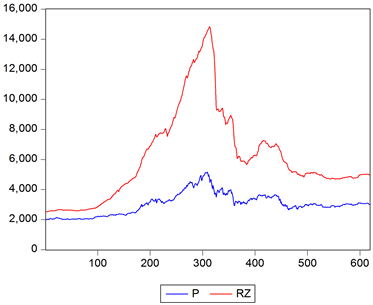# 基于GARCH模型的融资融券对我国股市的影响研究A Research about Effects Margin Transaction Has on the Stock Market in China Based on GARCH Model

DOI: 10.12677/FIN.2018.81005, PDF, HTML, XML, 下载: 1,004  浏览: 2,947  科研立项经费支持

Abstract: Select 619 logarithmic ratio of Shanghai Composite Index and balances of financing and securities loan during the period between 12th March, 2014 and 14th September, 2016 as sample data. Firstly, AIC and SC criteria are used to confirm the lag item numbers of GARCH model. Then, we introduce dummy variables as the partition of bull market and bear market, establish GARCH (1,1) model including dummy variables and analyze the effects that the system of margin transaction has on stock market volatility. The results indicate that: 1) Financing transaction in both bull market and bear market increases stock market volatility and the amplified influence that financing transaction has on the volatility in the bear market is more significant than that in the bull market. 2) Securities trading increases the volatility in the bull market while restrains that in the bear market. Finally, we combine the temporal systems, policies and investors’ mentality and from statistical analysis, we draw a conclusion: In China, financing business and securities business develop unevenly and the scale of financing business is far larger than that of securities business. Thus, in China, financing business exerts more important effects on the fluctuation of the stock market than securities business.

1. 引言

2. 文献综述

3. 实证分析

3.1. 样本选取和变量定义

Vol表示“股市波动性”；RZ表示“融资余额(亿元)”；RQ表示“融券余额(亿元)”

3.2. 模型的建立和估计

3.2.1. 正态性检验

3.2.2. 平稳性检验

3.2.3. 相关性检验

3.2.4. ARCH效应检验

$Vo{l}_{t}=C+{\epsilon }_{t}$

3.2.5. GARCH模型的建立及估计

Engle在1982年首次提出了ARCH模型(自回归条件异方差模型)该模型可用来刻画波动率的聚集特征及收益率的厚尾特性。但由于其自相关系数高并缓慢衰减的特征，ARCH(q)往往需要较大的q才能刻画资产收益率波动率的变化。T.Bollerslev于1986年提出了广义ARCH(GARCH)模型进行改进，它能以非常简约的形式刻画波动率的高阶自相关性以及资产收益率的厚尾特征。  GARCH模型的一般表达式是含有q个ARCH项和p个GARCH项，即GARCH(p,q)：

${\sigma }_{t}^{2}={\alpha }_{0}+{\lambda }_{1}{\sigma }_{t-1}^{2}+\cdots +{\lambda }_{p}{\sigma }_{t-p}^{2}+{\alpha }_{1}{u}_{t-1}^{2}+\cdots +{\alpha }_{q}{u}_{t-p}^{2}$

GARCH(p,q)应满足的条件是 ${\alpha }_{0}>0,\text{\hspace{0.17em}}{\alpha }_{i}\ge 0,\text{\hspace{0.17em}}i=1,2,\cdots ,q,\text{\hspace{0.17em}}{\lambda }_{i}\ge 0,\text{\hspace{0.17em}}i=1,2,\cdots ,p,\text{\hspace{0.17em}}0\le {\sum }_{i=1}^{q}{\alpha }_{i}+{\sum }_{i=1}^{p}{\lambda }_{i}<1$

${D}_{t}=\left\{\begin{array}{l}0,\text{ }2015.06.12以前，\\ 1,\text{ }2015.06.12以后.\end{array}$

$\left\{\begin{array}{l}Vo{l}_{t}=C+{\epsilon }_{t}\\ {\epsilon }_{t}={\sigma }_{t}{u}_{t},{u}_{t}~iid\text{\hspace{0.17em}}Ν\left(0,1\right)\\ {\sigma }_{t}^{2}={\alpha }_{1}+\alpha {\epsilon }_{t-1}^{2}+\beta {\sigma }_{t-1}^{2}+{\alpha }_{2}{D}_{t}+{\beta }_{1}R{Z}_{t}+{\beta }_{2}R{Q}_{t}+{\beta }_{3}\left({D}_{t}R{Z}_{t}\right)+{\beta }_{4}\left({D}_{t}R{Q}_{t}\right)\end{array}$ (1)

${\sigma }_{t}^{2}={\alpha }_{1}+\alpha {\epsilon }_{t-1}^{2}+\beta {\sigma }_{t-1}^{2}+{\beta }_{1}R{Z}_{t}+{\beta }_{2}R{Q}_{t}$ (2)

${\sigma }_{t}^{2}=\left({\alpha }_{1}+{\alpha }_{2}\right)+\alpha {\epsilon }_{t-1}^{2}+\beta {\sigma }_{t-1}^{2}+\left({\beta }_{1}+{\beta }_{3}\right)R{Z}_{t}+\left({\beta }_{2}+{\beta }_{4}\right)R{Q}_{t}$ (3)Table 5. The regression results of the GARCH(1,1) model

3.2.6. GARCH模型的结果解释Table 7. Correlation analysis of the Shanghai composite index with the total amount of financing and short sellingFigure 1. Monthly variation tendency of balance of financingFigure 2. Daily variation tendency ofShanghai Composite index and balance of financing

3.3. 总结与政策性建议

3.3.1. 总结

1) 从融资交易的角度来说，在牛市时(2014.3.12~2015.6.12)，融资交易增加了股市的波动性。这是因为融资交易带来了资金杠杆，使得盈亏放大，人们在股市行情较好的情况下，纷纷进行融资，加大杠杆，从而带来了极大的杠杆风险。而在这次熊市时(2015.6.15~2016)，融资交易也增加了股市的波动性，且这种波动性和在牛市是非对称的，模型结果显示，在熊市时，融资交易能给股市带来更大的波动性。

2) 从融券交易的角度来说，在牛市时，融券交易加剧了市场的波动性。而在熊市时，融券交易能够对股市波动性起到抑制的作用。然而，现实情况是在2015~2016年的熊市时，股市波动性依然很大，上证综指一路暴跌，这也从侧面说明了，在现阶段，我国的融券制度对股市波动性的抑制作用十分有限。

3) 从两融交易总体来看，两融业务开展时间较短，2010年3月31日才刚刚起步，我国的融资融券交易还存在着很多问题。第一，融券业务和融资业务发展极为不均衡，其中融资业务占主导地位，所以两融业务对股市波动性的影响，主要是融资业务对股市波动性的影响，也就是说在短期内，两融业务会增强对股市波动性，且在熊市时，两融业务对股市波动性的放大作用会比牛市时大。第二，目前融资融券业务的门槛依然很高，个人账户的开户的基本条件是在过去20个交易日中，账户日均资产满50万元。但是我国大部分个人投资者持股市值集中在10万元以下，截至2016年2月，我国持股市值在50万元以上个人投资者仅占总数的4.83% (数据来源：中证登，申万宏源研究)。第三，我国的个人投资者习惯于单边做多投资思维。投资者习惯于投资其看涨的股票，而不习惯于做空交易。

3.3.2. 政策性建议

1) 有序推进融资融券标的股票的扩容。之前我们分析到，我国融券规模较小的原因之一是证券公司券源有限，推进标的股票的扩容，可以促进融券业务的进行，避免投资者想融券却无券可融的局面。在一个健全的融资融券制度中，是否应该融券的标准应该是是否看跌股票价格而不是是否有券可融。

2) 适当降低融资融券的门槛。目前，我国的融资融券门槛是50万元。这个门槛对于个人投资者过高，而个人投资者占股市总投资者的99.71% (截至2016年2月)。应该鼓励证券公司根据自己客户的实际情况，设定自己的门槛，但是同时也要对证券公司设置的门槛实施强有力的监控，避免像2014年~2015年牛市期间那样，投资者以过高的资金杠杆进行融资的情况。

3) 加大对融资融券交易理念的宣传和教育。我国大部分投资者仍属于非理性投资者，应该鼓励证券公司对客户进行普及性的融资融券理念的教育，尽量使客户的投资观念趋于理性，这样做有利于投资者进行融资融券并用的双向投资，而非仅仅执着于融资的单向投资，能够很好地改善两融业务发展极不均衡的情况。

  Lintner, J. (1971) The Effect of Short Selling and Margin Requirements in Perfect Capital Markets. Journal of Financial and Quantitative Analysis, 6, 1173-1195. https://doi.org/10.2307/2329855  Kim, W.H. (1996) Short-Sales Restrictions and Volatility the case of the Stock Exchange of Singapore. Pacific-Basin Finance Journal, 4, 377-391. https://doi.org/10.1016/S0927-538X(96)00018-2  Bris, A., Goerzmann, W.N. and Zhu, N. (2007) Efficiency and the Bear: Short Sales and Markets around the World. The Journal of Finance, 62, 1029-1079. https://doi.org/10.1111/j.1540-6261.2007.01230.x  Sobaci, C., Sensoy, A. and Erturk, M. (2014) Impact of Short Selling Activity on Market Dynamics: Evidence from An Emerging Market. Journal of Financial Stability, 15, 53-62. https://doi.org/10.1016/j.jfs.2014.08.010  Bohl, M.T., Reher, G. and Wilfling, B. (2016) Short Selling Constraints and Stock Returns Volatility: Empirical Evidence from German Stock Market. Economical Modelling, 58, 159-166. https://doi.org/10.1016/j.econmod.2016.05.025  王虎, 朱贵宇. 论融资融券对中国股市波动性的影响[J]. 商业时代, 2012(7): 64-65.  冯玉梅, 陈璇, 张玲. 融资融券交易对我国股市波动性的影响研究——基于融资融券转常规前后的比较检验[J].山东社会科学, 2015(2): 130-134.  吴国平, 谷慎. 融资融券交易对我国股市波动性影响的实证研究[J]. 价格理论与实践, 2015(10): 105-107.  张兰兰. 论融资融券制度对我国股市波动性的影响——基于VAR模型分析[J]. 时代金融, 2016(6): 138-142.  倪伟佳. 我国融资融券业务对股票波动性的影响分析——基于TGARCH模型的长期研究[J]. 中国证券期货, 2013(7): 28-30.  姜近勇, 潘冠中. 金融计量经济学[M]. 北京: 中国财政经济出版社, 2011(7): 119-130.  胡忆文. 融资融券对我国股市波动性影响的实证研究[J]. 财经论丛, 2017(4): 55-60.